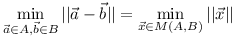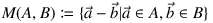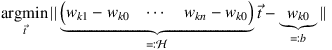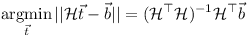# A divide-and-conquer implementation of the GJK algorithm

The Gilbert-Johnson-Keerthi (GJK) algorithm is a method for collision detection of convex polyhedra. The method is used in multiple rigid body simulations for computer games.

Given:

• a set of points defining the convex polyhedron A
• a set of points defining the convex polyhedron B

The algorithm returns:

• the distance of the polyhedra
• two closest points
• the two simplices (convex subsets of up to 4 points in 3 dimensions) of A and B containing the two closest points

An n-dimensional simplex is the convex hull of n+1 points as shown in the figure below.The algorithm makes use of the fact, that the distance between two sets A and B is the distance of the Minkowski difference to the origin:whereThe GJK algorithm iteratively updates a simplex until the closest point to the origin is found.

The algorithm iterates using support points. Given a set M and a vector d, the support point is defined as the furthest point of M in direction d:The GJK algorithm detects the two closest points of A and B as follows:

1. Choose any point m in M(A,B) in the Minkowski difference of A and B.
2. Set the initial simplex w0 to w0={m}.
3. Let d be the closest point of wk to the origin.
4. If d s(wk, -d)>=d s(M,-d) then return d.
5. Set w’k+1=wk∪{s(M,-d)}
6. Set wk+1 to the smallest simplex in w’k+1 still containing s(M,-d).
7. Continue with step 3.

Note that step 3 requires finding the closest point of the simplex wk to the origin. Most implementations of the GJK algorithm seem to use the following approach:

• Check if the origin is contained in the 3D simplex.
• If not, check whether the origin is near one of the 4 surfaces of the simplex.
• If the origin is not in the Delaunay region of any surface, check whether the origin is near one of the 6 edges.
• If not in a Delaunay region of an edge, find the closest point of the 4 points.

A much more compact implementation can be obtained using a divide-and-conquer approach:

• Let wk={wk0,wk1,…,wkn}
• Solve the least squares equation system• If all ti>=0 and t1+t2+…+tn<=1 (or n=0), then wk0+Ht is the closest point.
• Otherwise take the closest point of all sub-simplices with n-1 dimensions using the approach above (i.e. recursion).

Note that this approach will visit each edge up to two times, and each point up to three times. The performance is not optimal, but it makes for a much more concise implementation.

The least squares solution is:Here follows an implementation in Scheme (GNU Guile). By using pairs of points from A and B instead of the Minkowski difference, one can keep track of the information required to determine the pair of closest points of A and B (instead of the closest point of M to the origin).

Here an example of two colliding cuboids simulated using this implementation is shown:

Any feedback, comments, and suggestions are welcome.

Enjoy!

Update:

I noticed that Baraff just uses a separating plane algorithm to achieve the same!